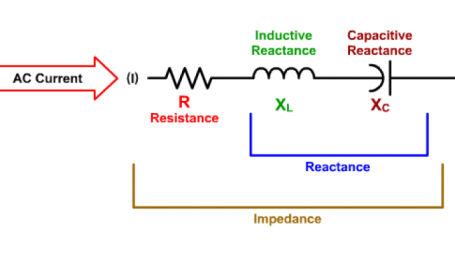Interactive

# Impedance in AC Circuits

Martech
Updated Jan 21, 2021

At the end of this lesson, you will be able to determine the total impedance of an AC circuit and apply Ohm’s Law to find other unknown values. • Describe a phasor diagram • Demonstrate the use of Ohm’s Law to calculate for unknowns in an AC circuit • Define impedance • Identify the steps to find impedance in a resistive/inductive series AC circuit • Identify the steps to find impedance in a resistive/capacitive series AC circuit • Identify the different steps needed to find impedance in a parallel AC circuit of resistance and reactance

;